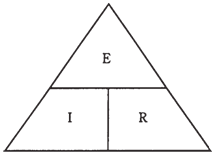## Ohm’s Law Assignment Help

Assignment Help: >> Electrical Engineering - Ohm’s Law

Ohm's Law

The interdependence between voltage, current, and resistance is one of the basic fundamental rules, or laws, in electrical circuits. It is termed as Ohm's Law, named after the scientist who supposedly first expressed it. Three formulas denote this law:

E = IR

I =E/R

R = E/I

You are required to remember the 1st one in order to derive the others. The simplest way to remember it is to learn abbreviations E for EMF or voltage, I for current and R for resistance, and then remember that they appear in the alphabetical order having equals sign after the E.

At times the 3 symbols are written in a triangle, which is shown in the Figure drawn below. To find the value of one, you cover it and read the positions of others.Figue--Ohm's Law triangle.

It is important to remember that you should use units of amperes, volts, and ohms in order for Ohm's Law to work right. If you use milliamperes, volts, and ohms or microamperes, kilovolts, and megohms you cannot expect to get right answers.

If the initial quantities are given in units other than volts, amperes, and ohms, you should convert to these units, and then calculate. After that, you can convert the units back to any unit you like, for instance, if you get 13,500,000 ohms as a calculated resistance, you may to say that it is 13.5 megohms.